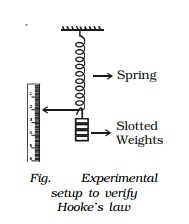Home | | Physics | Elasticity: Stress, strain Elastic limit, Hooke's law

# Elasticity: Stress, strain Elastic limit, Hooke's law

When an external force is applied on a body, which is not free to move, there will be a relative displacement of the particles. Due to the property of elasticity, the particles tend to regain their original position.

Elasticity

When an external force is applied on a body, which is not free to move, there will be a relative displacement of the particles. Due to the property of elasticity, the particles tend to regain their original position. The external forces may produce change in length, volume and shape of the body. This external force which produces these changes in the body is called deforming force. A body which experiences such a force is called deformed body. When the deforming force is removed, the body regains its original state due to the force developed within the body. This force is called restoring force. The property of a material to regain its original state when the deforming force is removed is called elasticity. The bodies which possess this property are called elastic bodies. Bodies which do not exhibit the property of elasticity are called plastic. The study of mechanical properties helps us to select the material for specific purposes. For example, springs are made of steel because steel is highly elastic.

Stress and strain

In a deformed body, restoring force is set up within the body which tends to bring the body back to the normal position. The magnitude of these restoring force depends upon the deformation caused. This restoring force per unit area of a deformed body is known as stress.

Stress = restoring force/ area N m-2

Its dimensional formula is ML-1T-2.

Due to the application of deforming force, length, volume or shape of a body changes. Or in other words, the body is said to be strained. Thus, strain produced in a body is defined as the ratio of change in dimension of a body to the original dimension.

Strain = change in dimension / original dimension

Strain is the ratio of two similar quantities. Therefore it has no unit.

Elastic limit

If an elastic material is stretched or compressed beyond a certain limit, it will not regain its original state and will remain deformed. The limit beyond which permanent deformation occurs is called the elastic limit.

Hooke's law

English Physicist Robert Hooke (1635 - 1703) in the year 1676 put forward the relation between the extension produced in a wire and the restoring force developed in it. The law formulated on the basis of this study is known as Hooke's law. According to Hooke's law, within the elastic limit, strain produced in a body is directly proportional to the stress that produces it.(i.e) stress α strain

Stress / Strain = a constant, known as modulus of elasticity.

Its unit is N m-2 and its dimensional formula is ML-1T-2

Experimental verification of Hooke's law

A spring is suspended from a rigid support as shown in the Fig.. A weight hanger and a light pointer is attached at its lower end such that the pointer can slide over a scale graduated in millimeters. The initial reading on the scale is noted. A slotted weight of m kg is added to the weight hanger and the pointer position is noted. The same procedure is repeated with every additional m kg weight. It will be observed that the extension of the spring is proportional to the weight. This verifies Hooke's law.

Study Material, Lecturing Notes, Assignment, Reference, Wiki description explanation, brief detail
11th 12th std standard Class Physics sciense Higher secondary school College Notes : Elasticity: Stress, strain Elastic limit, Hooke's law |